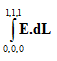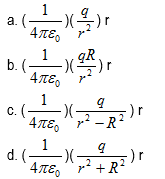Objective questions on Electric Potential for Jee Main and Advanced

Paragraph Type of Question
(A) Electric potential at any point x, y, z in the space is given V = 4x2 - 3x
Question 1
Find the electric field at any point (x, y, z)
a. (8x - 3)i
b. -(8x - 3)i
c. -8xi
d. 8xi

Question 2
Equipotential surface in the region are
a. planes parallel to X-Y plane
b. planes parallel to Y-Z plane
c. planes parallel to X-Z plane
d. none of the above

Question 3
Find the force experienced by a charge particle q at point (1, 1, 1)
a. -11qi
b. 11qi
c. 11qi + j
d. 11qi – j

Question 4
Find the value ofa. -1 volt
b. 0 volt
c. -2 volt
d. 1 volt

(B) A spherical shell of radius R is carries a uniform surface charge q. Take the reference point at ∞
Question 5
Find the electric field at r>R .Here is the r is the unit vector across radial directionQuestion 6
Find the electric field at r<R
a. (1/4πε0) (q/R2)
b. (1/4πε0) (q/r2)
c. zero
d. (1/4πε0) (q/r2 + R2)

Question 7
Find the potential at r>R
a. (1/4πε0) (q/r)
b. (1/4πε0) (q/r +R)
c. (1/4πε0) (q/r - R)
d. none of the above

Question 8
Find the potential at r<R
a. (1/4πε0) (q/r)
b. (1/4πε0) (q/R)
c. (1/4πε0) (q/R - r)
d. none of the above

Question 9
If I placed an second uniformly charge shell (Q) at radius R'>R, will the value of potential change at r<R
a. increases
b. decreases
c. constant
d. none of the above

Question 10
What will the electric field at r<R
a. (1/4πε0) (q/r2)
b. (1/4πε0) (q/R2)
c. zero
d. (1/4πε0) (q/r + R)

Single Answer type question
Question 11
A point charge q is located at (2, 3, 3) in xyz coordinate. Find the potential differences between A and B
A = (2, 3, 3)
B = (-2, 3, 3)
1. q/ 4πε0
2.  1/16πε0
3.  3q/16πε0
4.  none of the above

Question 12
Two equally charges are placed on the x-axis at (-a, 0) and (a, 0). Charge is q and mass is m. They are released from rest. Find their velocities when they are 4a apart
a. q √(1/πε0m)
b. (q/4) √(1/πε0m)
c. q/πε0m
d. q/4πε0m

Question 13
A point charge q1 = +2µC is placed at the origin of coordinates. A second charge
q2 = -3µC is placed on the y-axis at y = 100cm. At what point on the y-axis, potential is zero
a. y = -200cm
b. y = 40cm
c. y = 200cm
d. none of the above